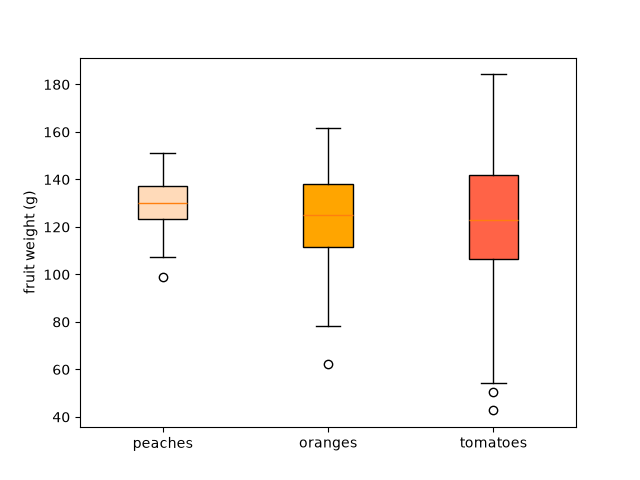# Box plots with custom fill colors#

This plot illustrates how to create two types of box plots (rectangular and notched), and how to fill them with custom colors by accessing the properties of the artists of the box plots. Additionally, the labels parameter is used to provide x-tick labels for each sample.

A good general reference on boxplots and their history can be found here: http://vita.had.co.nz/papers/boxplots.pdf

import matplotlib.pyplot as plt
import numpy as np

# Random test data
np.random.seed(19680801)
all_data = [np.random.normal(0, std, size=100) for std in range(1, 4)]
labels = ['x1', 'x2', 'x3']

fig, (ax1, ax2) = plt.subplots(nrows=1, ncols=2, figsize=(9, 4))

# rectangular box plot
bplot1 = ax1.boxplot(all_data,
vert=True,  # vertical box alignment
patch_artist=True,  # fill with color
labels=labels)  # will be used to label x-ticks
ax1.set_title('Rectangular box plot')

# notch shape box plot
bplot2 = ax2.boxplot(all_data,
notch=True,  # notch shape
vert=True,  # vertical box alignment
patch_artist=True,  # fill with color
labels=labels)  # will be used to label x-ticks
ax2.set_title('Notched box plot')

# fill with colors
colors = ['pink', 'lightblue', 'lightgreen']
for bplot in (bplot1, bplot2):
for patch, color in zip(bplot['boxes'], colors):
patch.set_facecolor(color)

for ax in [ax1, ax2]:
ax.yaxis.grid(True)
ax.set_xlabel('Three separate samples')
ax.set_ylabel('Observed values')

plt.show()References

The use of the following functions, methods, classes and modules is shown in this example:

Gallery generated by Sphinx-Gallery